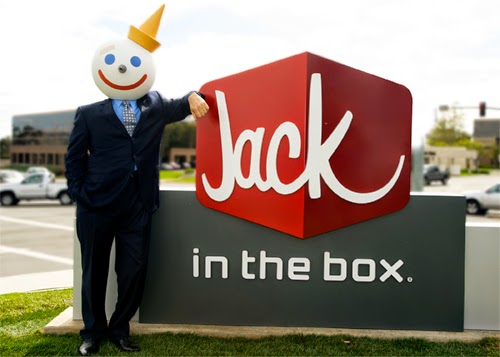## Saturday, May 31, 2014

### 33 | Jack in the Box, Robert Peterson and Leonard CommaJack = 10+1+3+11 = 25 | In = 9+14 = 23 | The 20+8+5 = 33 | Box = 2+15+24 = 41
Jack in the Box was founded in 1951, by Robert O. Peterson, in San Diego, California.  It is headquartered there today.  The company claims to operate approximately 2,200 locations.  In 2013, the year the NASDAQ reports, it is said the company employed 22,000 people.  Notice the master number 22 in both 22,000 and 2,200.
• Master = 4+1+1+2+5+9 = 22
• Robert = 9+6+2+5+9+2 = 33
• Oscar = 6+1+3+1+9 = 20
• Peterson = 7+5+2+5+9+1+6+5 = 40
• Robert Oscar Peterson = 33+20+40 = 93
Notice the first name of Robert, and the "33" numerology- this is a common trait in CEOs, who are often named Michael, Robert, Stephen, Leonard, Howard, and other "33 names".  The total sum of 93 is the number that represents the religion of Thelema, and a number that was central to the 9/11 and '93 WTC attacks.  On the date of 9/11/2001, it was Flight 93 that crashed in the "Keystone" State with 33 passengers and 11 crew.
• Keystone = 2+5+7+1+2+6+5+5 = 33
In the case of Jack in the Box, the only other CEO of the company other than Robert O. Peterson, is current CEO Leonard A. Comma.  As stated above, the name Leonard has '33' numerology as well.
• Leonard = 3+5+6+5+1+9+4 = 33
• Comma = 3+6+4+4+1 = 18
As for the company name, the red log with the word Jack, it also has connections to 33, with the number sequences 311 and 113.  Notice the product of 3x11 and 11x3 is 33.  In history, from World War I, to Star Wars to 9/11, even up to Fukushima, the numbers 311, 113 and 33 have been central in these events.  Now let us decode "Jack" and see.
• Jack = 1+1+3+11
• Jack = 11311
• Do you see the sequence of 113 and 311?
It has been reported that Disney and Pixar code the numbers 113 and 311 into their movies.  The first animator for Disney films, and one of Walt Disney's closest friends, was Jack Kinney.
• Kinney = 2+9+5+5+5+7 = 33
• Jack = 1+1+3+11
Jack in the Box the phrase has interesting numerology as well.
• Jack = 10+1+3+11 = 25
• In = 9+14 = 23
• The = 20+8+5 = 33
• Box = 2+15+24 = 41
• Jack In = 25+23 = 48
• The Box = 33+41 = 74
• Jack in the Box = 48+74 = 122